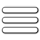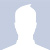ATP EducationThe Secure way of LearningWelcome! Guest

CBSE And NCERT Solutions:

# NCERT Solutions for Class 11 Mathematics english Medium 1. Sets

Page 1 of 7

## 1. Sets

### Exercise 1.1

(i) The collection of all the months of a year beginning with the letter J.
(ii) The collection of ten most talented writers of India.
(iii) A team of eleven best-cricket batsmen of the world.
(iv) The collection of all boys in your class.
(v) The collection of all natural numbers less than 100.
(vi) A collection of novels written by the writer Munshi Prem Chand.
(vii) The collection of all even integers.

(viii) The collection of questions in this Chapter.
(ix) A collection of most dangerous animals of the world.

(i) Members of this collection are:

January, June, July

∴ this collection is well-defined and hence, It is a set.

(ii) A writer may be most talented for one person but may not be so for other.

∴ this collection is unclear term and not well-define, so it is not a set.

(iii) A batsman may be best to one person and may not be so for other. Hence the term 'best' is unclear and not well-define. So it is not a set.

(iv) The members of this collection is definite as a class room.

∴ This collection is well-defined and so it is a set.

(v) The collection of all natural numbers less than 100 is well-define as it can be kept in a set like 1, 2, 3, 4, .......... 99.

∴  this collection is a set.

(vi) All novels writen by the writer Munshi Prem Chand can be kept in a group and this collection is well-define. So it is a set.

(vii) All even number like 2, 4, 6, 8, ............. can be kept in a group.

∴  this collection is well-define and so it is a set.

(viii) Questions in this chapter are clearly can be kept in well-define group. Therefore this collection is set.

(ix)  Any animal may be the most dangerous for someone but may not be for other. Therefore this term is unclear and not well-define. So it is not a set.

Q2. Let A = {1, 2, 3, 4, 5, 6}. Insert the appropriate symbol ∈ or ∉ in the blank spaces:
(i)   5. . . . . . A           (ii)  8 . . . . . .  A                      (iii)   0. . . . . . A
(iv) 4. . . . . . A           (v)  2. . . . . . . A                      (vi) 10. . . . . . A

(i)  5 ∈  A

(ii) 8 ∉ A

(iii) 0 ∉ A

(iv) 4 ∈  A

(v) 2 ∈  A

(vi)  10 ∉ A

Q3. Write the following sets in roster form:

(i) A = {x : x is an integer and –3 ≤ x < 7}

Solution:

A = {-3, -2, -1, 0, 1, 2, 3, 4, 5, 6 }

(ii) B = {x : x is a natural number less than 6}

Solution:

B = {1, 2, 3, 4, 5}

(iii) C = {x : x is a two-digit natural number such that the sum of its digits is 8}

Solution:

C = (17, 26, 35, 44, 53, 62, 71, 80}

(iv) D = {x : x is a prime number which is divisor of 60}

Solution:

Divisor of 60 ⇒ 2, 3, 4, 5, 6, 12, 15, 20, 30, 60

but looking for prime number, which are 2, 3, 5

∴ D = {2, 3, 5}
(v) E = The set of all letters in the word TRIGONOMETRY

Solution:

E = {T, R, I, G, O, N, M, E, Y}

Note: repeated letter can only be kept once.

(vi) F = The set of all letters in the word BETTER.

F = {B, E, T, R}

Q4. Write the following sets in the set-builder form :

(i) (3, 6, 9, 12}

Solution:

A = {x : x is a natural number multiple of 3 and x < 15}

Or

A = { x : x = 3n, n ∈ N and 1≤ n ≤4}

(ii) {2,4,8,16,32}

Solution:

B = { x : x = 2n , n ∈ N and 1≤ n ≤5}

(iii) {5, 25, 125, 625}

Solution:

C = {x : x = 5n , n ∈ N and 1≤ n ≤4}

(iv) {2, 4, 6, . . .}

Solution:

D = {x : x is an even natural number }

(v) {1,4,9, . . .,100}

Solution:

E = {x : x = n2, n ∈ N and n < 11  }

Or

E = {x : x = n2, n ∈ N and 1≤ ​n ≤10  }

Or

E = {x : x = n2, where n is the first 10 natural number }

Q5. List all the elements of the following sets :

(i) A = {x : x is an odd natural number}

Solution:

A = {1, 3, 5, 7, 9, ....... }

 (ii) B = {x : x is an integer, - 1 < x < 9 } 2 2

B = {0, 1, 2, 3, 4}

(iii) C = {x : x is an integer, x2 ≤ 4}

Solution:

x  √4

x ≤ ±2

the number between -2 to 2.

C = {-2, -1, 0, 1, 2}

(iv) D = {x : x is a letter in the word “LOYAL”}

Solution:

D = {L, O, Y, A}

(v) E = {x : x is a month of a year not having 31 days}

Solution:

E = {February, April, June, September, November}

(vi) F = {x : x is a consonant in the English alphabet which precedes k }.

Solution:

consonant befor Letter 'k'

F = {b, c, d, f, g, h, j}

Q6. Match each of the set on the left in the roster form with the same set on the right described in set-builder form:

 (i) {1, 2, 3, 6} (a) {x : x is a prime number and a divisor of 6} (ii) {2, 3} (b)  {x : x is an odd natural number less than 10} (iii) {M,A,T,H,E,I,C,S} (c) {x : x is natural number and divisor of 6} (iv) {1, 3, 5, 7, 9} (d) {x : x is a letter of the word MATHEMATICS}.

Solution:

(i)  ------------------ (c)

(ii) ------------------ (a)

(iii) ------------------ (d)

(iv) ------------------ (b)

www.atpeducation.com

www.atpeducation.com

Page 1 of 7

## Topic Lists:

##### NCERT Solutions⇒Class 11th ⇒ Mathematics ⇒ Chapter 1. Sets:

See other sub-topics of this chapter:Roshan Class X says: "6"Shadab Khan Class X says: "make fast all science pages geography "Shivam Bajpai All Class says: "ये पेज under construction क्युं है .plz fix this prob..."Krishan Class X says: "this very good website i really appreciate which provide no cost education to all medium classes"Vimal Class XI says: "Not able to find the content....as instructed."Kamini Class X says: "every chepter is imcomplete....this side is not useful"Ashok Swami All Class says: "10th science ka Lesson-8 ka page no.5 kab take under construction rahega please improve it."Rishabh Gupta Class XI says: "how can i understand difference between permutation and combination word problem"Rishabh Gupta Class XI says: "i want to learn parts of speech"KASHIF ALI Class VIII says: "Please update all the syllabus of class 8"